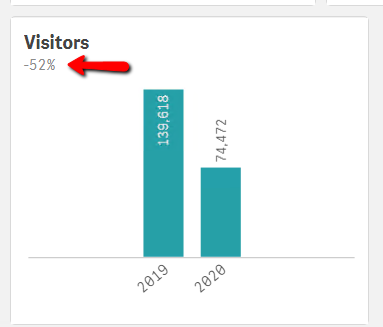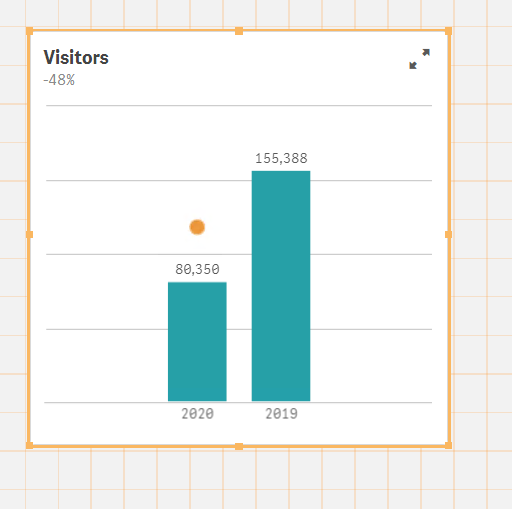# App Development

Announcements
Now Live: Qlik Sense SaaS Simplified Authoring – Analytics Creation for Everyone: READ DETAILS
cancel
Showing results for
Did you mean:Creator

## MTD/PMTD bar chart

Hi,

I created a bar chart which displays current year and previous year  MTD.

I need to show the difference between the 2 values. Couldn’t figure out a way add this to the chart, so I added it to the title.

Anyone has an idea?

The dimension is Year

The bar expression:

Sum({<[Calendar.Year] ={">=\$(vPrevYear)<=\$(vMaxYear_1)"},[Calendar.MonthNum] = {'\$(vMaxMonth_1)'}, [Calendar.Day] = {"<=\$(vMaxDay_1)"}>}Customers_Count)

The difference expression:

(\$(v_CustCount_MTD_Dashboard)

/

\$(v_CustCount_PMTD_Dashboard) )

-1,'#,##0%')

Thanks!Labels (2)

• ### MTD

1 Solution

Accepted SolutionsLuminary Alumni

Set Analysis happens before the data gets calculated over the dimensions. You can't do value of Dimension A vs Dimension B, while the Dimension is in the chart, unless you use inter-record functions, like Above() or change the model.

Here is how you can do it using Above().

``````Sum({<[Calendar.Year] ={">=\$(vPrevYear)<=\$(vMaxYear_1)"},[Calendar.MonthNum] = {'\$(vMaxMonth_1)'}, [Calendar.Day] = {"<=\$(vMaxDay_1)"}>}Customers_Count)

/

Above(Sum({<[Calendar.Year] ={">=\$(vPrevYear)<=\$(vMaxYear_1)"},[Calendar.MonthNum] = {'\$(vMaxMonth_1)'}, [Calendar.Day] = {"<=\$(vMaxDay_1)"}>}Customers_Count))``````

You should probably include year 2018 as well, so 2019 has a comparison value. If you uncheck 'Include zero Values' in the Add-Ons section, it will hide 2018 from the chart and only show 2019 & 2020, which the comparison %.

2 RepliesLuminary Alumni

Set Analysis happens before the data gets calculated over the dimensions. You can't do value of Dimension A vs Dimension B, while the Dimension is in the chart, unless you use inter-record functions, like Above() or change the model.

Here is how you can do it using Above().

``````Sum({<[Calendar.Year] ={">=\$(vPrevYear)<=\$(vMaxYear_1)"},[Calendar.MonthNum] = {'\$(vMaxMonth_1)'}, [Calendar.Day] = {"<=\$(vMaxDay_1)"}>}Customers_Count)

/

Above(Sum({<[Calendar.Year] ={">=\$(vPrevYear)<=\$(vMaxYear_1)"},[Calendar.MonthNum] = {'\$(vMaxMonth_1)'}, [Calendar.Day] = {"<=\$(vMaxDay_1)"}>}Customers_Count))``````

You should probably include year 2018 as well, so 2019 has a comparison value. If you uncheck 'Include zero Values' in the Add-Ons section, it will hide 2018 from the chart and only show 2019 & 2020, which the comparison %.Creator
Author

It works!  Thank you very much!